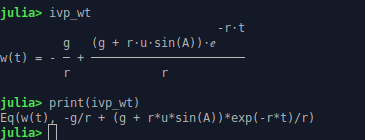# What is alternative command besides `print(ivp_vt)` to looks like when only typing `ivp_vt` in REPL?

I want to make
`print(ivp_vt)` shown like when I type `ivp_vt`, but the problem is if I type `print(ivp_vt)` there is this bugging Eq at front and the format becomes ugly as well, the fraction should be in two rows instead of just one.

What is the command besides `print()` that can make me print:

``````ivp_vt
ivp_wt
``````

when running one Julia code in REPL to show beautifully?

this is the code:

``````using SymPy, Plots
@syms t, g, r, A, u
@syms v()
@syms w()

# Computing IVP with SymPy
diffeqv = Eq(v(t).diff(t), -r*v(t)); string(diffeqv)
diffeqw = Eq(w(t).diff(t), -g -r*w(t)); string(diffeqw)

# To solve the ODE, pass it and the function to solve with dsolve.
vt = dsolve(diffeqv, v(t))
wt = dsolve(diffeqw, w(t))

sol_vt = rhs(vt)
sol_wt = rhs(wt)

# To solve the Initial Value Problem, initial condition v(0) = u cos (A)
ivp_vt = dsolve(diffeqv, v(t), ics = Dict(v(0) => u*cos(A) ))
ivp_wt = dsolve(diffeqw, w(t), ics = Dict(w(0) => u*sin(A) ))

# Manual way
# Find the constant C₁
c1 = free_symbols(vt)
# To solve the Initial Value Problem, initial condition v(0) = u cos (A)
C1 = solve(sol_vt(t=>0) ~ u*cos(A), c1)
#ivp_vt = sol_vt(c1=>C1)

println("Modeling with First Order Equations with Julia")
println("A baseball is thrown with the initial speed of u and initial angle of elevation is A")
println("v(t) is the horizontal component of the velocity of the thrown baseball")
println("w(t) is the vertical component of the velocity of the thrown baseball")
println("x(t) is the horizontal coordinate of the thrown baseball at time t")
println("y(t) is the vertical coordinate of the thrown baseball at time t")

println("")
println("The horizontal component of the velocity of a thrown baseball : ")
print(ivp_vt)
print(ivp_wt)
``````Maybe `sympy.pretty_print(ivp_wt)`?

1 Like

Yes that is what I am talking about. It looks like textbook on Julia REPL now

Turns out, SymPy alone with its future version is the only package needed for all Mathematics branches